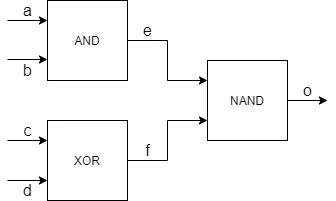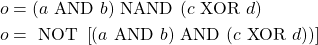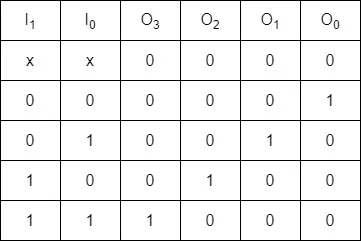# Logic Design - Combinational Logic Examples [Verilog][Edit of Image1]

## Introduction

Hey it's a me again @drifter1!

Today we continue with the Logic Design series on Verilog to get into Combinational Logic Examples, as promised in the previous part. The first example will be on the different implementation styles for combinational logic, whilst the next ones will be examples of common circuits (encoder, decoder and multiplexer).

So, without further ado, let's dive straight into it!

## One Circuit - Four Implementations

Consider the logic circuit shown below.Let's implement the circuit in Verilog using:

1. assign statement (data flow)
2. combinational always block
3. gate primitives (structural)
4. user-defined primitives (truth table)

### The module

The first three implementations share the module definition part of the Verilog HDL code. More specifically, because the circuit has 4 inputs and 1 output, and those are by default of type wire, the module outline can be defined as follows:

``````module circuit1 (output o, input a, b, c, d);
/* main module */
endmodule``````

### 1. Assign Statement

Using the assign statement syntax of Verilog, which is used for data flow modeling, its possible to implement the functionality of the circuit with just one line of code:

``assign o = ~((a & b) & (c ^ d));``
This might seem a little bit complicated, but that's just because Verilog doesn't have an NAND operator, and so the following reformation is performed:so that the NAND operator turns into an AND operator followed by a logic negation (NOT) on the result.

### 2. Combinational Always Block

The assign statement can be easily replicated using a combinational always block. The keyword assign is simply removed from that line of code and put inside of an always block. Of course, all of the four inputs turn into conditions of the always block.

``````always @ (a | b | c | d) begin
o = ~((a & b) & (c ^ d));
end``````
The begin and end keywords are optional in this case, as only one statement is part of the assign block.

### 3. Gate Primitives

Because of Verilog's gate primitives, its easy to implement the logic circuit by instantianting three such primitives. Two new signals of type wire have to be declared with names e and f. Those signals will be the output of the AND and XOR gate correspondingly, whilst the output of the NAND gate will be driven towards the module output.

The code for all that is simply:

``````wire e, f;
and (e, a, b);
xor (f, c, d);
nand (o, e, f);``````
Refresh note: the output of gate primitives is always connected first.

### 4. User-Defined Primitives

Lastly, in order to implement the circuit as a truth table, a primitive needs to be declared, instead of a module. Let's first specify it using the complete truth table:

``````primitive circuit1(o, a, b, c, d);
table
// a b c d   o
0 0 0 0 : 1
0 0 0 1 : 1
0 0 1 0 : 1
0 0 1 1 : 1
0 1 0 0 : 1
0 1 0 1 : 1
0 1 1 0 : 1
0 1 1 1 : 1
1 0 0 0 : 1
1 0 0 1 : 1
1 0 1 0 : 1
1 0 1 1 : 1
1 1 0 0 : 1
1 1 0 1 : 0
1 1 1 0 : 0
1 1 1 1 : 1
endtable
endprimitive``````
As you can see only two cases lead to a logical zero (0) in the output. These cases are known as max-terms of a logic function, and using them its possible to shorten the code using '?':
``````table
// a b c d   o
1 1 0 1 : 0
1 1 1 0 : 0
1 1 0 0 : 1
1 1 1 1 : 1
0 ? ? ? : 1
? 0 ? ? : 1
endtable``````
I'm far of an expert in such things, but I think that I've covered all the cases using these rows.

## Encoder

An encoder takes in 2n-bit inputs and produces n-bit outputs. The most common implementation is known as "one-hot", where a legal combination of values has only a single high (1) bit and all the others low (0). Sometimes the opposite known as "one-cold" is also used, where only a single bit is low (0) and the others are high (1).

For example, the truth table of a 4-to-2 one-hot encoder could be defined as:This functionality can be implemented using OR gates, where each of the output bits is the result of an OR operation on two of the input bits. But, that way its impossible to guarantee the "one-hot" encoding. Therefore, the most commonly used encoders are priority encoders, which lead to a more complicated logic circuit.

Either way, an encoder can be implemented in Verilog using control blocks, and so inside of an always block using nested if-else statements or an case statement.

Using if-else statements, the 4-to-2 encoder mentioned in the example, can be implemented as follows:

``````module encoder_4to2 (output [1 : 0] o, input [3 : 0] i);
always @ (i) begin
if (i == 4'b0001) o = 2'b00;
else if (i == 4'b0010) o = 2'b01;
else if (i == 4'b0100) o = 2'b10;
else if (i == 4'b1000) o = 2'b11;
else o = 2'bxx;
end
endmodule``````

Using a case statement, the code inside of the always block changes into:

``````case (i)
4'b0001 : o = 2'b00;
4'b0010 : o = 2'b01;
4'b0100 : o = 2'b10;
4'b1000 : o = 2'b11;
default : o = 2'bxx;
endcase
``````

## Decoder

An decoder is the exact opposite of an encoder. The decoder takes in n-bits and outputs 2n-bits, again using either one-hot or one-cold encoding.

For example, the truth table for a 2-to-4 one-hot decoder looks as follows:In Verilog, the decoder can again implemented using an if-else or case control block. As such, a case statement implementation of a 2-to-4 decoder looks like this:

``````module decoder_2to4 (output [3 : 0] o, input [1 : 0] i);
always @ (i) begin
case (i)
2'b00 : o = 4'b0001;
2'b01 : o = 4'b0010;
2'b10 : o = 4'b0100;
2'b11 : o = 4'b1000;
default : o = 4'b0000;
endcase
end
endmodule``````

It's also possible to implement the decoder using a left shift on '1' by an amount equal to the input. This can be done in a single line of code using an assign statement as follows:

``assign o = 1 << i;``
Of course this generates inferior circuitry, and should be avoided. But, it's worth mentioning it!

## Multiplexer

Lastly, another very commonly used circuit is the multiplexer.

A multiplexer has a maximum of 2n input lines, n selection lines and a single output line. Depending on the value on the selection lines, the corresponding input is passed to the output.

On the gate-level side of things, the multiplexer is basically implemented using AND, OR and NOT gates.

For example, a 4x1 multiplexer has the following truth table:or can be written as a boolean function as follows:Though, multiplexers can yet again be implemented either using an if-else or an case statement.

For example, using the case statement approach, an 4x1 multiplexer is defined as follows:

``````module mux_4to1 (output o, input [1 : 0] s, input [3 : 0] i);
always @ (i, s) begin
case (s)
2'b00 : o = i;
2'b01 : o = i;
2'b10 : o = i;
2'b11 : o = i;
default : o = 0;
endcase
end
endmodule``````

Small multiplexers can also be implemented using the assign statement and conditional operator.

As such, a 2x1 multiplexer could be defined as:

``````module mux_2to1 (output o, input s, input [1 : 0] i);
assign o = s ? i : i;
endmodule``````

## Final words | Next up

And this is actually it for today's post!

Next time we will get into how Sequential Logic is defined using Verilog...

See Ya!Keep on drifting!

H2
H3
H4
3 columns
2 columns
1 column
Join the conversation now